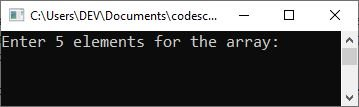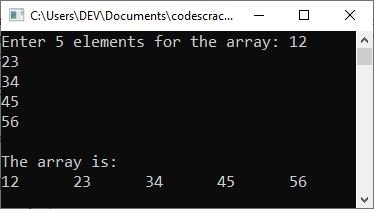# C++ One-Dimensional Array

This post will teach you about one-dimensional arrays in C++. So, without further ado, let's get started.

A group of elements that all share the same data type and name is what makes up a one-dimensional array. The common name of each element as well as its own distinct index are used to identify each individual element.

## Declare a One-Dimensional Array in C++

The simplest form of an array is a one-dimensional array. The array itself is given a name, and its elements are referred to by their subscripts. In C++, a one-dimensional array is denoted as follows:

`type name[size];`

where "type" is the data type, "name" is the name of the one-dimensional array, and "size" is the number of elements that the array can hold. As an example:

`int arr;`

declares an array "arr" that can store up to 5 elements of type "int."

## Initialize a One-Dimensional Array in C++

Here is the general form to initialize values in a one-dimensional array in C++.

`data_type array_name[array_size] = {comma_separated_element_list};`

Here is an example of declaring and initializing values for the array name arr of type int, which contains 10 elements.

`int arr = {1, 2, 3, 4, 5, 6, 7, 8, 9, 10};`

If you are interested in viewing the programs that were created on a 1-D array, it is recommended that you read the article titled "one-dimensional array programs." On the other hand, after I finish explaining this point, I'll give you some examples to help you better grasp the concept and for your own convenience.

Here are some C++ example programs that demonstrate one-dimensional arrays. The following is the first example program:

```#include<iostream>
using namespace std;
int main()
{
int arr = {1, 2, 3, 4, 5};
for(int i=0; i<5; i++)
{
cout<<arr[i]<<endl;
}

cout<<endl;
return 0;
}```

The output of this C++ program is as follows:

```1
2
3
4
5```

That is, the first element (1) is stored at "arr," similarly, the second element (2) is stored at "arr," and so on. Therefore, using just the "for" loop with its variable running from 0 to 4, I printed the elements of each array on the output console.

Now let me modify the above program to print the value of each element along with its indexes.

```#include<iostream>
using namespace std;
int main()
{
int i, arr = {10, 20, 23, 43, 50};

for(i=0; i<5; i++)
cout<<"arr["<<i<<"] = "<<arr[i]<<endl;

cout<<endl;
return 0;
}```

Now the output should be:

```arr = 10
arr = 20
arr = 23
arr = 43
arr = 50```

Now is the time to again modify the above program to allow the user to enter the elements for the array:

```#include<iostream>
using namespace std;
int main()
{
int i, arr;

cout<<"Enter 5 elements for the array: ";
for(i=0; i<5; i++)
cin>>arr[i];

cout<<"\nThe array is:\n";
for(i=0; i<5; i++)
cout<<arr[i]<<"\t";

cout<<endl;
return 0;
}```

The following snapshot shows the initial output produced by the above program:Now provide the input. That is, type the first number and press ENTER, then the second number and press ENTER again. Provide five inputs as array elements and press the ENTER key to see the following output:Let me make one more change to the program. The following program allows the user to specify the array's size as well as its elements, and then prints the elements to the output console.

```#include<iostream>
using namespace std;
int main()
{
int i, tot;

cout<<"Enter the size for the array: ";
cin>>tot;
int arr[tot];

cout<<"\nEnter "<<tot<<" elements for the array:\n";
for(i=0; i<tot; i++)
{
cout<<"arr["<<i<<"] = ";
cin>>arr[i];
}

cout<<"\nThe array is:\n";
for(i=0; i<tot; i++)
cout<<arr[i]<<"  ";

cout<<endl;
return 0;
}```

The screenshot below shows a sample run of the above program with the following user inputs: 8 as size and 10, 20, 30, 40, 50, 60, 70, and 80 as array elements.#### More Examples on 1D Array

C++ Quiz

« Previous Tutorial Next Tutorial »